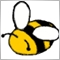# AES256 encode+decode493

Hi all,

this is the procedure I'm trying to accomplish :

• RAW_STRING : is a string from input
• this string is converted into a uchar array (considering the \0)
• encrypt the uchar array using AES256 method (with a constant and proper-sized key)
• represent a Hex array from the encrypted data (saved to ENCODED_STRING)

then

• an ENCODED_STRING is retrieved from input
• convert this string into a uchar array (Alain's HexToArray() method)
• decrypt it using AES256 (and exactly same key used above)
• convert back the decoded uchar array into string (this should give back the RAW_STRING)

but my code fails to regenerate the initial string. strangely enough it regenerates the RAW_string with some gibberish data attached to its end.

```const string constant_key = "ABCDEFGHIJKLMNOPQRSTUVWXYZ123456"; // 32 letters
uchar key[];
StringToCharArray(constant_key, key, 0, StringLen(constant_key));

string RAW_STR = "initial text from input";

// Encryption :
uchar src[], encrypted[];
StringToCharArray(RAW_STR, src, 0, StringLen(RAW_STR));
ResetLastError();
if(CryptEncode(CRYPT_AES256, src, key, encrypted)==0) { Print("ERROR [code:",_LastError,"]"); return; }
string ENCODED = "";
for(int i=0; i<ArraySize(encrypted); i++) ENCODED += StringFormat("%.2X", encrypted[i]);
Print("ENCODED string :\t", ENCODED);

// Decryption (in another MQL program) :
input string Decrypt_me = ""; // a string from input (which is ENCODED actually)
uchar source[], decrypted[];
ArrayResize(source, StringLen(Decrypt_me)/2);
HexToArray(Decrypt_me, source);
ResetLastError();
if(CryptDecode(CRYPT_AES256, source, key, decrypted)==0) { Print("ERROR [CODE:",_LastError,"]"); return; }
string DECODED = CharArrayToString(decrypted);
Print("DECODED string :\t", DECODED); // this is where sometimes RAW_STR is shown, sometimes not.

// Alain's hex string to uchar array : (modified for no error check)
#define HEXCHAR_TO_DECCHAR(h)  (h<=57 ? (h-48) : (h-55))
void HexToArray(string str, uchar &arr[])
{
uchar tc[];
StringToCharArray(str,tc);

int i=0, j=0;
for(i=0; i<StringLen(str); i+=2)
{
uchar tmpchr=(HEXCHAR_TO_DECCHAR(tc[i])<<4)+HEXCHAR_TO_DECCHAR(tc[i+1]);
arr[j]=tmpchr;
j++;
}
}```493

LoL
a few minutes after posting I see the problem.
```#define HEXCHAR_TO_DECCHAR(h)  (h<=57 ? (h-48) : (h-55))
void HexToArray(string str, uchar &arr[])
{
uchar tc[];
StringToCharArray(str, tc, 0, StringLen(str));

int i=0, j=0;
for(i=0; i<StringLen(str); i+=2)
{
uchar tmpchr=(HEXCHAR_TO_DECCHAR(tc[i])<<4)+HEXCHAR_TO_DECCHAR(tc[i+1]);
arr[j]=tmpchr;
j++;
}
}```

Mods, feel free to delete this thread. I found my answer, thanks to me :)
but maybe someone could benefit from it.

To title the process better :
Decrypting a NULL terminated string (retrieved from input), which is actually a Hex representation of an uchar array (encrypted data)

To add comments, please log in or register